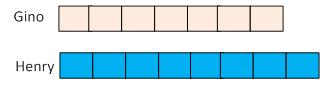### Sample Problem

Henry hadmore money than Gino. After Gino spent 50% of his money, he had \$24 less than Gino. How much money did Henry have left?

dollars

#### Solution

Henry hadmore money than Gino. So,

Set Gino's money as 7 units and Henry's money as (7 + 1 = 8) units. Then,8 units × (100% – 50%) = 4 units.

Henry had 4 units left.

Then, (7 units – 4 units) = 24

3 units = 24

1 unit = 24 ÷ 3 = 8

Thus, Henry's money left = 4 units = 4 × 8 = \$32.00